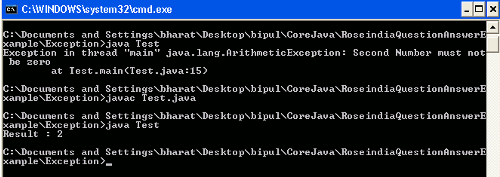# Java Throw Built-in Exception

In this section we are discussing about throwing of built-in exceptions in Java.

In this section we are discussing about throwing of built-in exceptions in Java.

## Java Throw Built-in Exception

In this section we are discussing about throwing of built-in exceptions in Java.

In Java throw and throws keywords are used to throw an Exception. throw keyword throws an exception forcibly that may use the custom messages whereas the keyword throws is used to throw a possible exception.

Example

Here I am going to give a simple example which will demonstrate you about how to use throw keyword in Java to throw the exception. In this example I am trying to find a result after dividing the two numbers. When you will divide the two non-zero numbers the outcome of result will be what you had expected but if the denominator is non zero then outcome of result will be unexpected. In this case I want to throw an ArithmethicException with the specified message that specifies the denominator must not be zero. In this example I have created a class named Test.java where I created a reference of ArithmeticException and a method that returns the result after dividing the two numbers. In this method I have checked for the denominator number that if it is equals to zero then this method throws the exception. Then in the main() method created object of Test class and called the method created for dividing two numbers.

Source Code

Test.java

```public class Test
{
ArithmeticException ae;
public int  divide(int numerator, int denominator)
{
if(denominator == 0)
{
throw ae;
}
return numerator/denominator;
}
public static void main(String[] args)
{
Test test = new Test();
test.ae = new ArithmeticException("Second Number must not be zero");

//int result = test.divide(4, 0);
int result = test.divide(4,2);
System.out.println("Result : "+ result);
}
}```

Output

When you will execute the above Java class then the output will be as follows :But when you will call the method by passing two non-zero numbers as passed into the above Java Class Test.java then after executing this class output will be as follows :